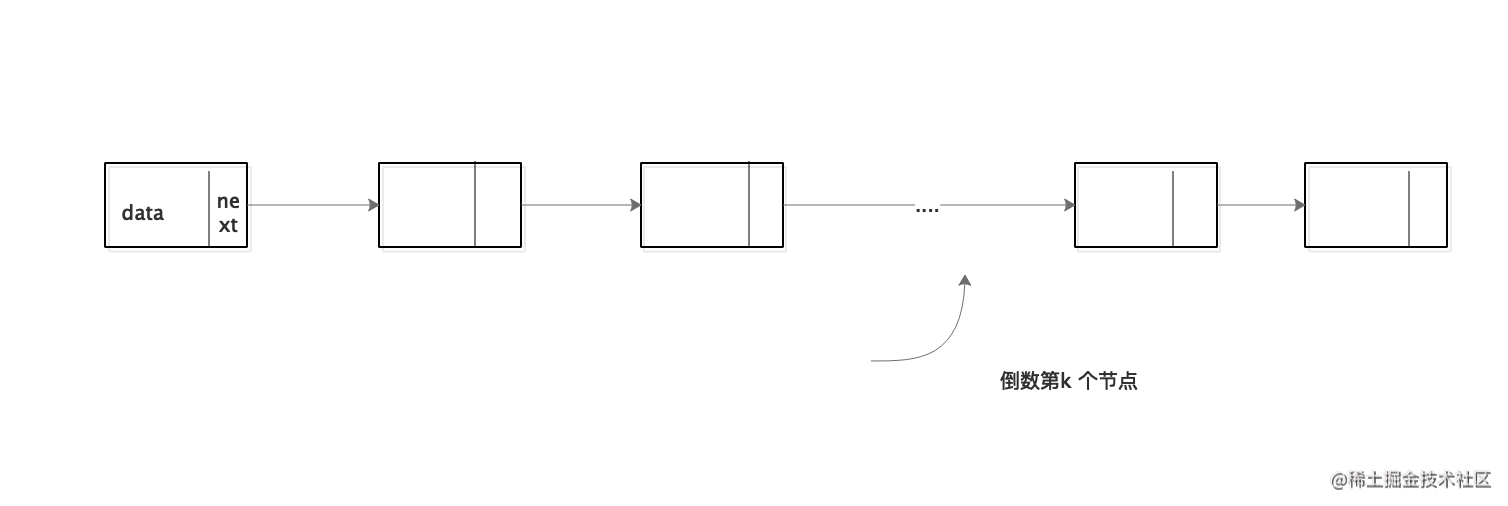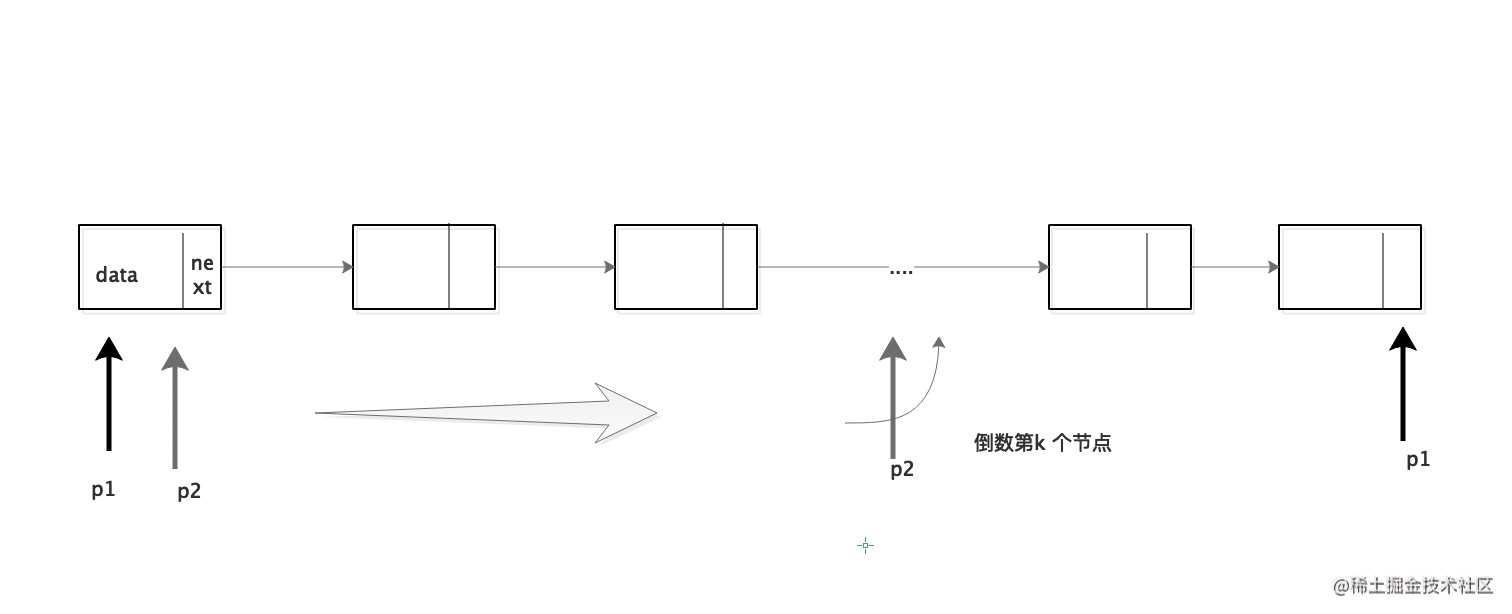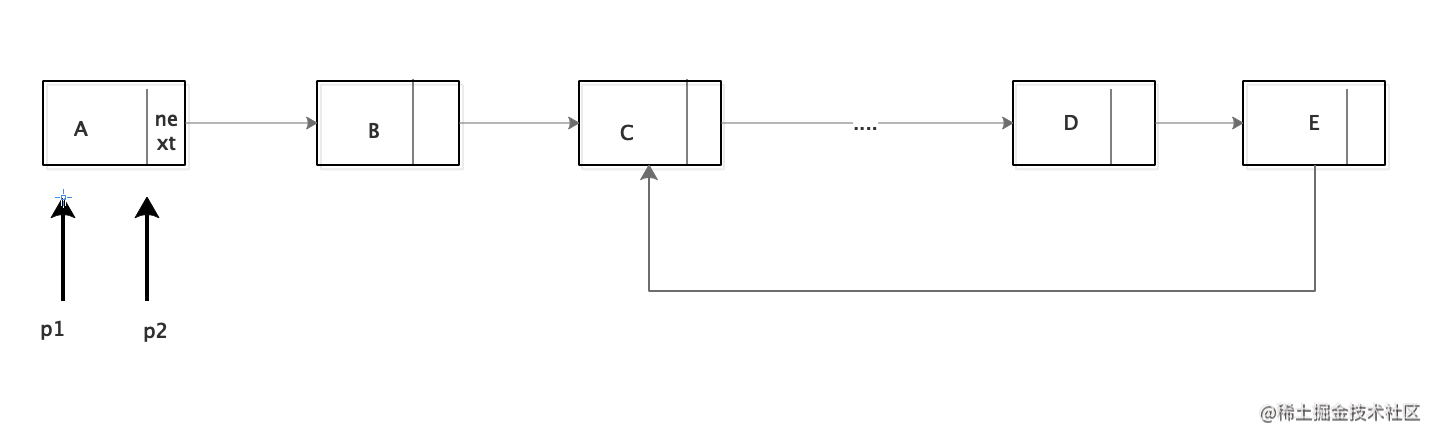# 链表算法经典题

``````/*链表*/struct ListNode {    int data;
struct ListNode *next;
struct ListNode *pre;
};

``````struct ListNode* constructList(void)
{   //头结点定义
//记录当前结点
struct ListNode *cur = NULL;
for (int i = 0; i < 8; i++) {
struct ListNode *node = malloc(sizeof(struct ListNode));
node->data = i;
//头结点为空,新结点即为头结点
} else {//当前结点的next为新结点
cur ->next = node;
}
//设置当前结点为新结点
cur = node;
}
}

``````struct ListNode* constructCycleList(void)
{
//头结点定义
//记录当前尾结点
struct ListNode *cur = NULL;
//环结点入口
struct ListNode *cycle = NULL;

for (int i = 0; i < 8; i++) {
struct ListNode *node = malloc(sizeof(struct ListNode));
node->data = i;
if (i == 3) {//第4个为环入口
cycle = node;
}
//头结点为空,新结点即为头结点
} else {//当前结点的next为新结点
cur->next = node;
}
//设置当前结点为新结点
cur = node;
if (i == 7) {
cur->next = cycle;
}
}
}

### 1. 链表的倒数第K个节点``````struct ListNode* findKth(struct ListNode *head, int k)
{
int i = 0;
while (cur!=NULL&&i++<k) {
cur = cur->next;
}
while (cur!=NULL) {
now =now->next;
cur =cur->next;
}
return now;
}

### 2.从尾到头打印链表（递归和非递归）

``````struct ListNode* reverseList(struct ListNode* head)
{
struct ListNode *reverseNode =reverseList(nextNode);
return reverseNode;
}

### 3.如何判断一个链表有环``````bool isLoopList(struct ListNode* head)
{
//使用快慢指针，慢指针每次向前一步，快指针每次两步
while (fastPoint != NULL && fastPoint->next != NULL) {
slowPoint = slowPoint->next;
fastPoint = fastPoint->next;
if (fastPoint->next != NULL) {
fastPoint = fastPoint->next;
//两指针相遇则有环
if (slowPoint == fastPoint) {
return true;
}
}
}
return false;
}

### 4.链表中环的大小

``````//首先找出相遇时候的环节点,然后再次到此节点则表示环大小
{
//使用快慢指针，慢指针每次向前一步，快指针每次两步
while (fastPoint != NULL && fastPoint->next != NULL) {
slowPoint = slowPoint->next;
fastPoint = fastPoint->next;
if (fastPoint->next != NULL) {
fastPoint = fastPoint->next;
//两指针相遇则有环
if (slowPoint == fastPoint) {
return slowPoint;
}
}
}
return NULL;
}

{

//node为空则代表链表无环
if (node == NULL) {
return 0;
}
int length = 1;
struct ListNode* currentNode = node->next;
//再次相遇则循环结束
while (currentNode != node) {
length++;
currentNode = currentNode->next;
}
printf("cycle length is %d",length);
return length;
}

### 5.链表中环的入口结点

``````struct ListNode* cycleListFirstNode(struct ListNode* head)
{